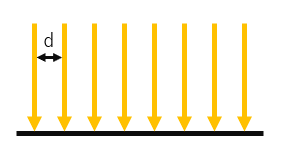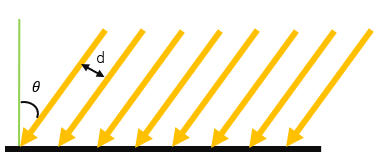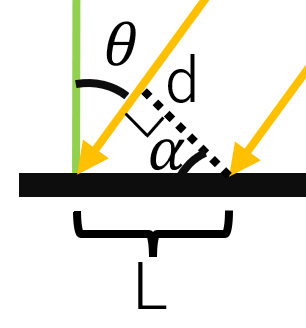#### 問題

Question. 非垂直光中的光線間的距離怎麼求解?a. 垂直光線b. 非垂直光線

#### 推導

$L = \frac{d}{\cos\theta}$$L = \frac{d}{cos \alpha}$，所以有

$L = \frac{d}{\cos\theta}$